# How important is Power Conversion Efficiency?

When it comes to power conversion in electronics, efficiency is one of the biggest challenges to become more competitive. As greatest the efficiency, the less energy waste and less warm the product is, which translates into a longer average life of electronic components.

The definition of efficiency is “the ratio of the useful work performed by a machine or a process to the total energy expended”. When talking about power converters, efficiency is a key parameter, as it is the ratio between the Input Power and Output Power.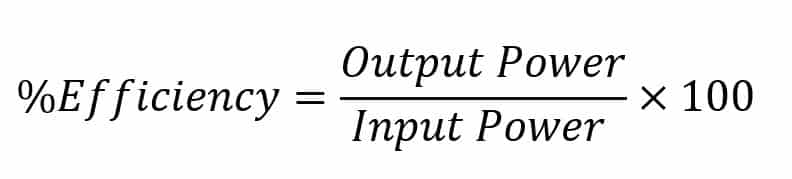As a practical example, Premium PSU’s CRS-1000-6494 (110Vin / 72Vout) at full load has an output voltage of 72Vdc and 13,9A and 110Vdc and 9,68A in the Input side. Calculating the efficiency is as easy as: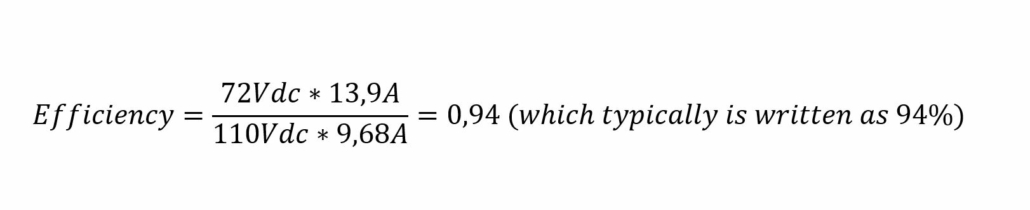In this case, 64W are “lost”: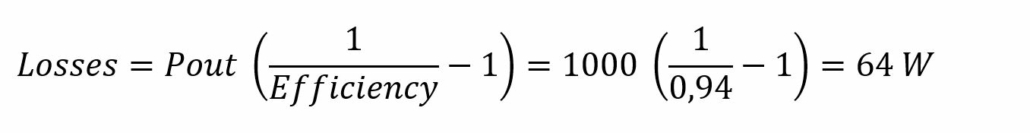## Why does the same converter have different efficiencies based on the model?

So, where does this energy go? All the losses of power converters are in the form of heat, and when we need to dissipate the heat using fans, this will affect the efficiency.

The heat is dissipated mainly from the PCB, MOSFETS, diodes and other electronic components such as resistors, transformers, inductors and capacitors.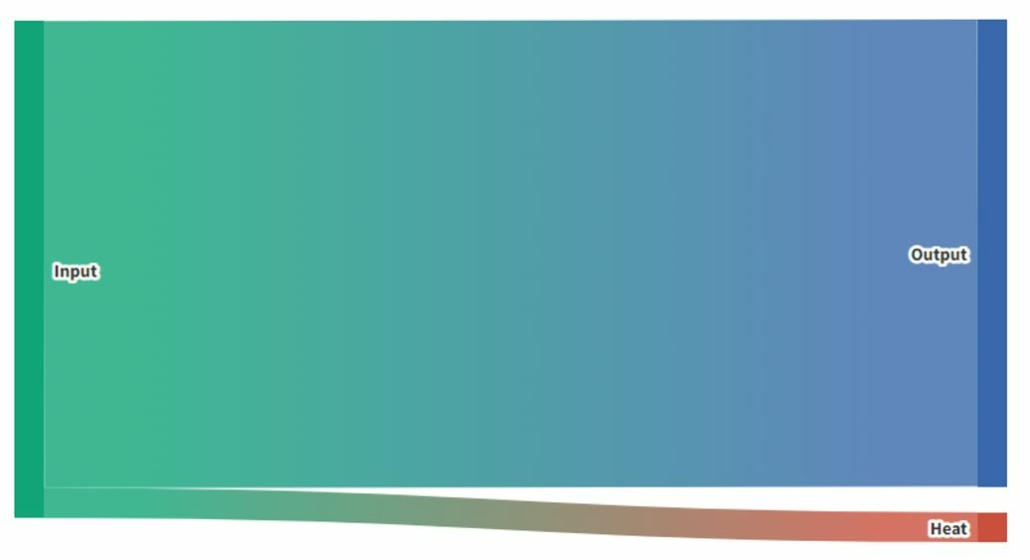Most of it can be described by Joule’s first Law: the heating power generated by an electrical conductor (applies also to the PCB tracks) equals the product of its resistance and the square of the current.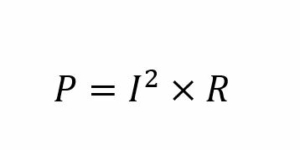This is why a CRS-1000 with higher voltages (and therefore lower currents) will dissipate less heat and, therefore, be more efficient.

Comparing Premium PSU’s DC/AC Inverter ODS-3000-7157 (110Vdc in) vs the ODS-3000-7153 (24Vdc In), the 24V model will have a maximum input current of over 160A vs 43A that can be found on the 110V model. This current difference impacts on the efficiency, which is 92% for the 110V model vs 89% for the 24Vdc model.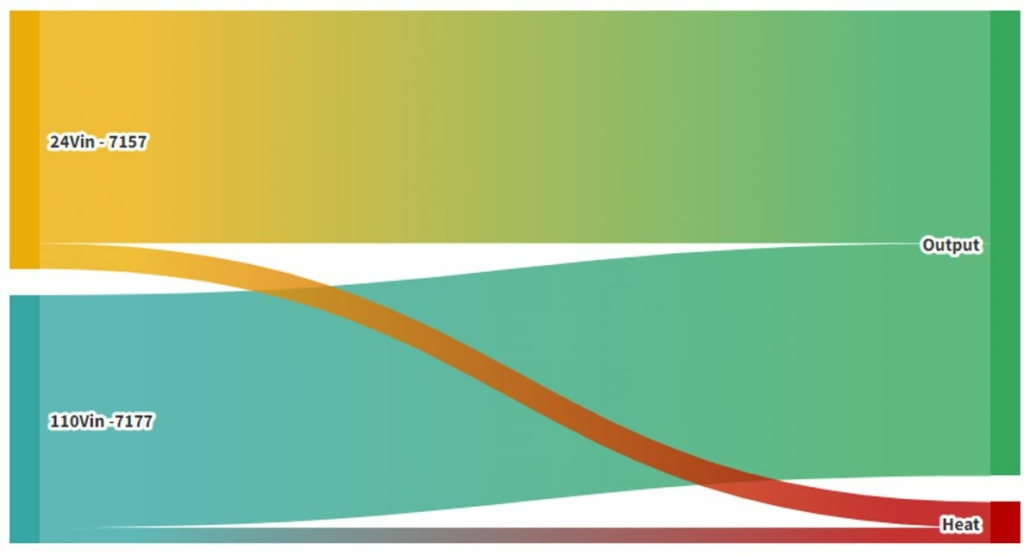In the graph above the energy transferred to heat is greater in the 24Vin model compared to the 110Vin.

## Why does efficiency matter?

Having a better energy efficiency will have an impact in cost, CO2 emissions and can affect the system life expectancy if the system uses batteries.  Let’s see how:

### Cost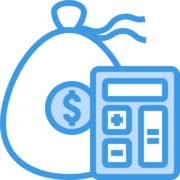Let’s imagine we use an inverter in a system, with a typical efficiency of 80%, which could be average in the market. Premium PSU’s typical inverter efficiencies range from 87 to 94% (90% average). What will be the impact of this 10% increased efficiency over 10 years? If the efficient inverter is 500€ more expensive, when will we have the ROI?

To make calculations easier, we will expect a constant energy cost. In this example we will consider a 3kW Inverter, with a 90% efficiency will have an Input Power of 3.330W, so basically 330W will be converted to heat.

If the efficiency is 80% instead, the heat generated will be 750W, which is 2,25 times more waste!

With an average cost of 0,2€/kWh, and considering that the inverters are working 12h a day for a year at 90% efficiency, the DC/AC inverter will have a loss of:While an 80% efficiency DC/AC inverter would cost: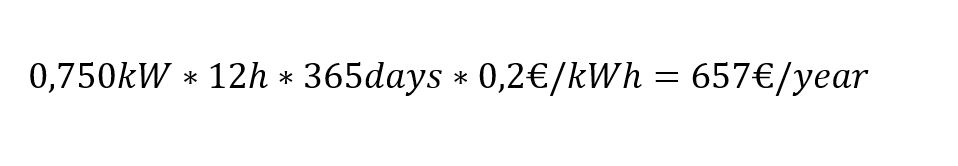With a difference of 368€/year the ROI to have a more efficient converter (500€ more expensive) will be just 1,35 years!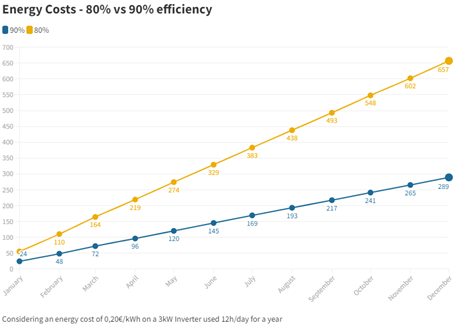### CO2

In 2020 the total US electricity generated approximately 385,55g of CO2 per kWh. Calculating the total CO2 difference that will be emitted to the atmosphere is very easy if we know that the heat losses are 520W less: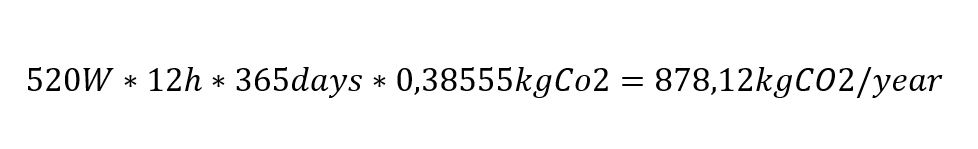This is equal of burning 393,7L of Fuel, or driving for 7.875km!

More than the distance from Premium PSU’s headquarters in Barcelona to the US or China.

(Considering an average consumption of 5L/100km and 2,34kgCO2/L)

### Battery life expectancy

And, what if this system is connected to batteries?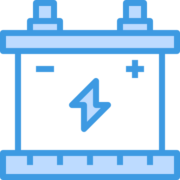With a more efficient inverter the battery time will increase, therefore the battery cycles needed will be reduced, not only saving energy but also increasing the system’s life expectancy.

Let’s take a 24Vdc 300Ah rated battery, and consider that we can deep discharge it and use the 300Ah every cycle… Also, we will consider that the capacity will not be reduced by charging and discharging it.

Using an 80% efficient inverter, the battery will be able to power the Inverter for 115 minutes vs the 131 minutes that we will get using a 90% efficient converter.

If the system works for 2 hours every day, with the more efficient converter, the battery will need to do 458 cycles, while the less efficient system will need 520 cycles, reducing its life expectancy.

As you can see, efficiency is not a minor feature to take into consideration when evaluating options in a power converter. For this reason at Premium PSU we keep on working to improve it in all our devices in order to make our customer’s applications not only competitive, but also the best option in the market.